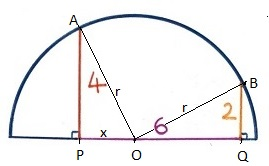# »  Solutions to puzzles in my VDARE.com monthly Diary

##February 2023

—————————In the Math Corner of my February 2023 Diary I asked for the area of this semicircle.

—————————

•  SolutionThis one's too easy. First, label up key points P, Q, A, and B. Then, since you know this is a semicircle, that bottom horizontal line is a diameter, so halfway along it is the circle's center O. Not knowing precisely where that is in relation to P and Q, let's just suppose it's a distance x to the right of P.

Construct radii, both of course length r, from O to A and B.

With two right-angled triangles there, sides (r, 4, x) and (r, 2, 6−x), you could now chew through the algebra, applying Pythagoras' Theorem to the two triangles APO and BQO, then eliminating r and solving for x.

It's hardly worth the trouble, though. Plainly x = 2 and the two triangles are congruent. So r² = 4² + 2², which is 20. A circle radius r has area πr², so our semicircle area is 10π.